# Texas Go Math Grade 8 Lesson 4.2 Answer Key Determining Slope and y-Intercept

Refer to our Texas Go Math Grade 8 Answer Key Pdf to score good marks in the exams. Test yourself by practicing the problems from Texas Go Math Grade 8 Lesson 4.2 Answer Key Determining Slope and y-Intercept.

## Texas Go Math Grade 8 Lesson 4.2 Answer Key Determining Slope and y-Intercept

Essential Question
How can you determine the slope and the y-intercept of a line?

Investigating Slope and y-intercept
The graph of every nonvertical line crosses the y-axis. The y-intercept is the y-coordinate of the point where the graph intersects the y-axis. The x-coordinate of this point is always 0.

The graph represents the linear equation y = –$$\frac{2}{3}$$ + 4.

Step 1
Find the slope of the line using the points (0, 4) and (-3, 6)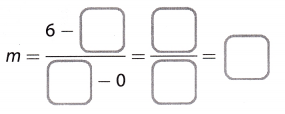Step 2
The line also contains the point (6, 0). What is the slope using (0, 4) and (6, 0)? Using (-3, 6) and (6, 0). What do you notice?

Step 3
Compare your answers in Steps 1 and 2 with the equation of the graphed line.

Step 4
Find the value of y when x = 0 using the equation y = –$$\frac{2}{3}$$x + 4. Describe the point on the graph that corresponds to this solution.

Step 5
Compare your answer in Step 3 with the equation of the line.

Find the slope and y-intercept of the line represented by each table.

Question 1.$$\frac{32-22}{4-2}$$ = 5 Finding the slope using given points by
$$\frac{42-32}{6-4}$$ = 5 slope (m) = (y2 – y1) ÷ (x2 – x1)
$$\frac{52-42}{8-6}$$ = 5
Slope(m) = $5$
Work backward from x = 2 to x = 0 Find the initial value when the value of x is 0
$$\frac{32-22}{4-2}$$ = $$\frac{10}{2}$$
x = 2 – 2 = 0 Subtract the difference of x and y from the first point
y = 22 – 10 = 12
y intercept (b) 12

Question 2.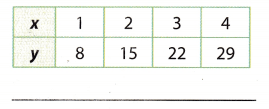The slope is calculated by:
m = $$\frac{y_{2}-y_{1}}{x_{2}-x_{1}}$$
Thus:So m = 7
To get the y-intercept (“b”) of the line, we need to include values of x and y in equation:
y = mx + b
Include values of x and y from one point, I will take the first one.
8 = 7 . 1 + b
b = 8 – 7
b = 1

Explore Activity 2

Deriving the Slope-intercept Form of an Equation

In the following Explore Activity, you will derive the slope-intercept form of an equation.

Step 1
Let L be a line with slope m and y-intercept b. Circle the point that must be on the line. Justify your choice.
(b, 0) (0, b) (0, m) (m, 0)

Step 2
Recall that slope is the ratio of change in y to change in x. Complete the equation for the slope m of the line using the y-intercept (0, b) and another point (x, y) on the line.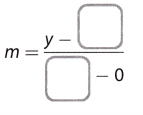Step 3
In an equation of a line, we often want y by itself on one side of the equation. Solve the equation from Step 2 for y.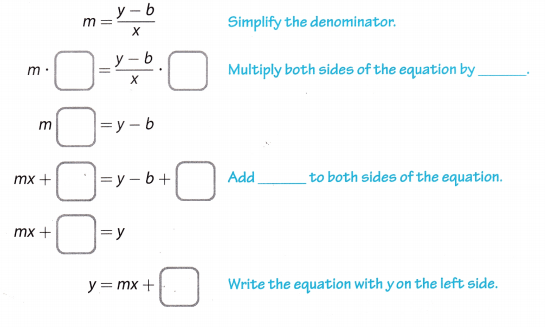Reflect

Question 3.
Critical Thinking Write the equation of a line with slope m that passes through the origin. Explain your reasoning.
y= x When the line passes through the origin, y intercept = 0 in
y = mx + b

Find the slope and y-intercept of the line in each graph. (Explore Activity 1)

Question 1.Slope m = ___ y-intercept b = ___
The The slope is calculated by:
m = $$\frac{y_{2}-y_{1}}{x_{2}-x_{1}}$$
We have point one (0, 1) and point two (2, -3). Include them into the calculation:
m = $$\frac{-3-1}{2-0}$$ = $$\frac{-4}{2}$$ = -2
m = -2

According to the equation y = mx + b we can calculate the y-intercept, that is b. Include the values of x and y from one point and the obtained slope value
y = mx + b
-1 = -2 . 0 + b
b = 1 – 0
b = 1

Question 2.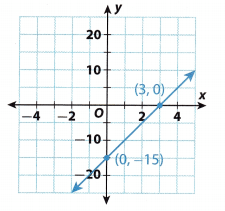Slope m = ___ y-intercept b = ___
The scope is calculated by:
m = $$\frac{y_{2}-y_{1}}{x_{2}-x_{1}}$$
We have point one (0,-15) and point two (3,0). Include them into the calculation:
m = $$\frac{0-(-15)}{3-0}$$ = $$\frac{0+15}{3}$$ = $$\frac{15}{3}$$ = 5
m = 5
According to the equation y = mx + b we can calculate the y-intercept, that is b. Include the values of x and y from one point and the obtained slope value.
y = mx + b
-15 = 5 . 0 + b
b = -15

Question 3.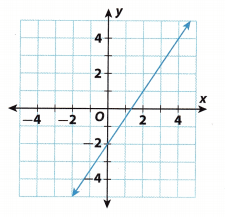Slope m = ___ y-intercept b = ___
The slope is calculated by:
m = $$\frac{y_{2}-y_{1}}{x_{2}-x_{1}}$$
First, read the points on the graph, the places where the graph intersects a certain values of the x and y axes. The first one is (0, -2) and the second one is (2, 1). Include them into the calculation:
m = $$\frac{1-(-2)}{2-0}$$ = $$\frac{1+2}{2}$$ = $$\frac{3}{2}$$
m = $$\frac{3}{2}$$

According to the equation y = mx + b we can calculate the y-intercept, that is b. Include the values of x and y from one point and the obtained slope value.
y = mx + b
-2 = $$\frac{3}{2}$$ . 0 + b
-2 = 0 + b
b = -2

Question 4.Slope m = ___ y-intercept b = ___
The slope is calculated by:
m = $$\frac{y_{2}-y_{1}}{x_{2}-x_{1}}$$
First, read the points on the graph, the places where the graph intersects a certain value of the x and y axes
The first one is (0,9) and the second one is (3,0). IncLude them into the calculation:
m = $$\frac{0-9}{3-0}$$ = $$\frac{-9}{3}$$ = -3
m = -3

According to the equation y = mx + b we can calculate the y-intercept, that is b. Include the values of x and y from one point and the obtained slope value.
y = mx + b
9 = -3 . 0 + b
9 = 0 + b
b = 9

Find the slope and y-intercept of the line represented by each table. (Example 1)

Question 5.slope m = ______y-intercept b = ______
m = $$\frac{y_{2}-y_{1}}{x_{2}-x_{1}}$$
Include values of x and y from the table, for each and next point:To get the y-intercept (“b”) of the line, we need to include values of x and y in equation:
y = mx + b
Include values of x and y from any point
1 = 3 . 0 + b
1 = 0 + b
b = 1

Question 6.The slope is calculated by:
m = $$\frac{y_{2}-y_{1}}{x_{2}-x_{1}}$$
Include values of x and y from the table, for each and next point:To get the y-intercept (“b”) of the line, we need to include values of x and y in equation:
y = mx + b
Include values of x and y from any point
140 = -4 . 0 + b
140 = 0 + b
b = 140

Essential Question Check-In

Question 7.
How can you determine the slope and the y-intercept of a line from a graph?
Choose any two points on the line from the graph and use it to find the slope.
Determine the point where the line crosses the y-axis to find y-intercept.

Question 8.
Some carpet cleaning costs are shown in the table. The relationship is linear. Find and interpret the rate of change and the initial value for this situation.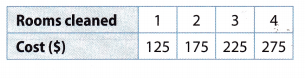It is already written in the task that the relationship is linear, so the slope (rate of change) can be found with only
two points.

The slope is calculated by:
m = $$\frac{y_{2}-y_{1}}{x_{2}-x_{1}}$$

First point is (1, 125) and the second point is (2, 175). Put those in calculation:
m = $$\frac{175-125}{2-1}$$ = $$\frac{50}{1}$$ = 50
The standard form of our Linear function is
y = mx – 1- b
We need to get the initial value of this function.
Take a look at the equation, compare the data we already have, and find what we need to get. Thus, the y intercept (”b”) is unknown.

Include the values of x, y and the slope in the equation and calculate:
y = mx + b
125 = 50 . 1 + b
125 = 50 + b
b = 125 – 50
b = 75

Question 9.
Make Predictions The total cost to pay for parking at a state park for the day and rent a paddleboat are shown.

a. Find the cost to park for a day and the hourly rate to rent a paddleboat.
Slope = $$\frac{29-17}{2-1}$$ = $$\frac{12}{1}$$ = 12 Finding the slope using any two given points by Slope(m) = (y2 – y2) ÷ (x2 – x1)
where
(x2, y2) = (2, 29) and (x1, y1) = (1, 17)
The hourly rent is $\$12$per hr Work backward from x = 1 to x = 0 Find the initial value when the value of x is 0 $$\frac{29-17}{2-1}$$ = $$\frac{12}{1}$$ x = 1 1 = 0 Subtract the difference of x and y from the first point. y = 17 – 12 = 5 The cost to park for a day is$\$5$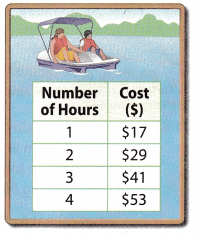b. What will Lin pay if she rents a paddleboat for 3.5 hours and splits the total cost with a friend? Explain.
Total Cost = 3.5(12) + 5 = 47 When Lin paddles for 3.5 hr
Lin’s Cost = $$\frac{47}{2}$$ = 23.5

Question 10.
Multi-Step Raymond’s parents will pay for him to take sailboard lessons during the summer. He can take half-hour group lessons or half-hour private lessons. The relationship between cost and number of lessons is linear.a. Find the rate of change and the initial value for the group lessons.
Slope = $$\frac{85-55}{2-1}$$ = $$\frac{30}{1}$$ = 30 Finding the slope using any two given points by
slope (m) = (y2 – y1) ÷ (x2 – x1)
Rate of change is $\$30$per lesson where (x2, y2) = (2, 85) and (x1, y1) = (1, 55) Work backward from x = 1 to x = 0 Find the initial value when the value of x is 0 $$\frac{85-55}{2-1}$$ = $$\frac{30}{1}$$ x = 1 – 1 = 0 Subtract the difference of x and y from the first point y = 55 – 30 = 25 The initial value of group lessons is$\$25$

b. Find the rate of change and the initial value for the private lessons.
Slope = $$\frac{125-75}{2-1}$$ = $$\frac{50}{1}$$ = 50 Finding the slope using any two given points by
slope (m) = (y2 – y1) ÷ (x2 – x1)
Rate of change is $\$50$per lesson where (x2, y2) = (2, 125) and (x1, y1) = (1, 75) Work backward from x = 1 to x = 0 Find the initial value when the value of x is 0 $$\frac{125-75}{2-1}$$ = $$\frac{50}{1}$$ x = 1 – 1 = 0 Subtract the difference of x and y from the first point y = 75 – 50 = 25 The initial value of group lessons is$\$25$

c. Compare and contrast the rates of change and the initial values.
The initial value for both types of lessons is same. Comparing results from part a and b.
Rate of change is higher for private lessons then group lessons.

Vocabulary Explain why each relationship is not linear.

Question 11.$$\frac{6.5-4.5}{2-1}$$ Finding the rate of change using given points by
slope (m) = (y2 – y1) ÷ (x2 – x1)
$$\frac{8.5-6.5}{3-2}$$ = 2
$$\frac{11.5-8.5}{4-3}$$ = 3
The rate of change is not constant, hence the relationship is not linear

Question 12.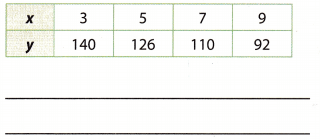Calculate the slope for each and next point.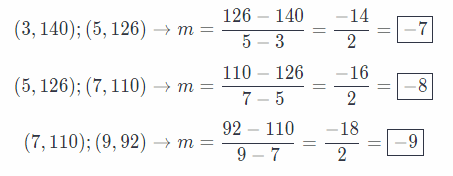Question 13.
Communicate Mathematical Ideas Describe the procedure you performed to derive the slope-intercept form of a linear equation. (Explore Activity 2)
Express the slope m between a random point (x, y) on the line and the point (0, b) where the line crosses the y
axis. Then solve the equation for y.

Texas Go Math Grade 8 Lesson 4.2 H.O.T. Focus On Higher Order Thinking Answer Key

Question 14.
Critique Reasoning Your teacher asked your class to describe a real-world situation in which the y-intercept is 100 and the slope is 5. Your partner gave the following description: My younger brother originally had 100 small building blocks, but he has lost 5 of them every month since.

a. What mistake did your partner make?
a. If the brother loses 5 blocks every month, the slope would be -5 and not 5.
When the initial value is decreasing, the slope is negative.

b. Describe a real-world situation that does match the situation.
Justify Reasoning John has a job parking cars. He earns a fixed weekly salary of $300 plus a fee of$5 for each car he parks. His potential earnings for a week are shown in the graph. At what point does John begin to earn more from fees than from his fixed salary? Justify your answer.After parking 60 cars, John’s earning become $600 double of his initial base salary of$300. Hence, after parking 61 cars, his earning from fee becomes more than his fixed salary.
$$\frac{300}{5}$$ = 60